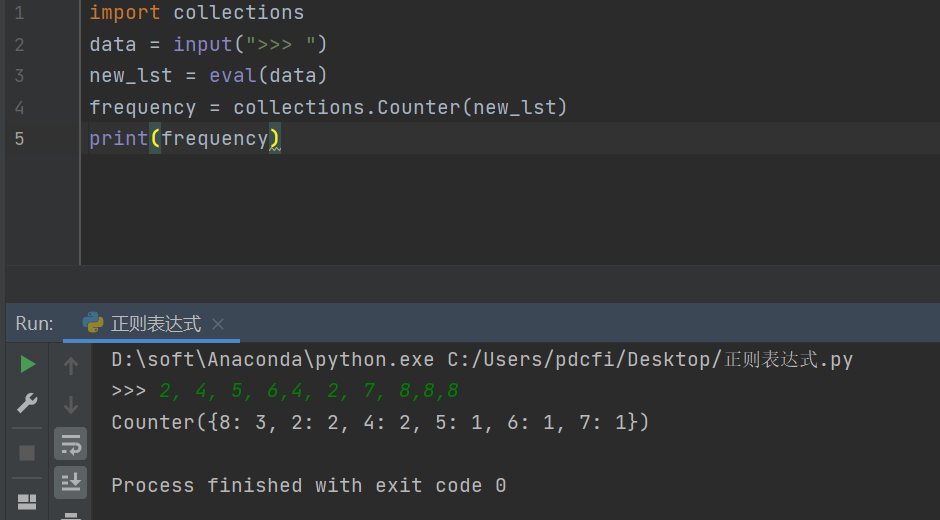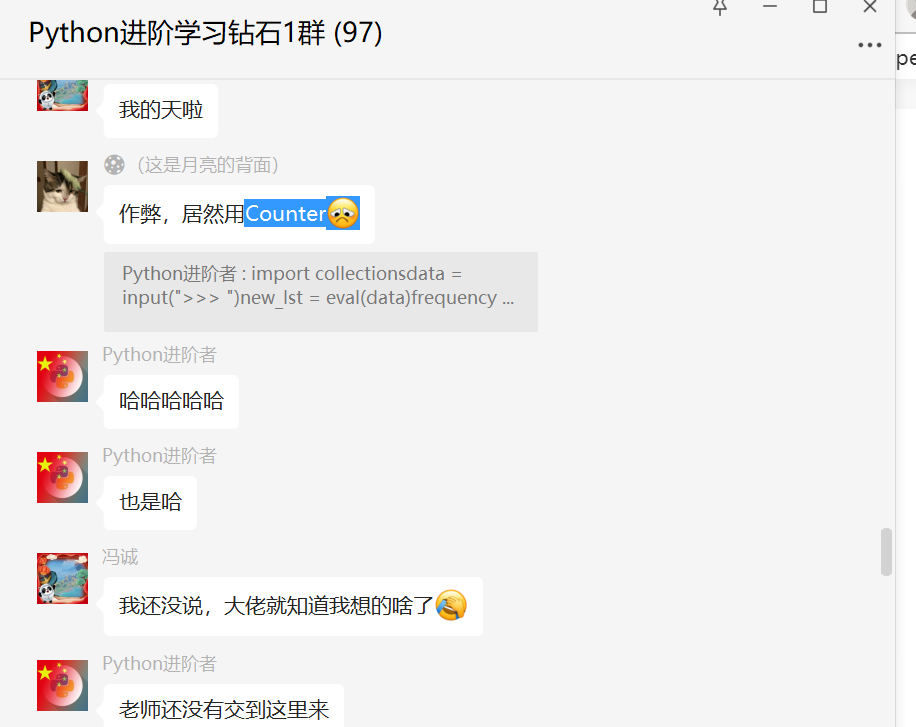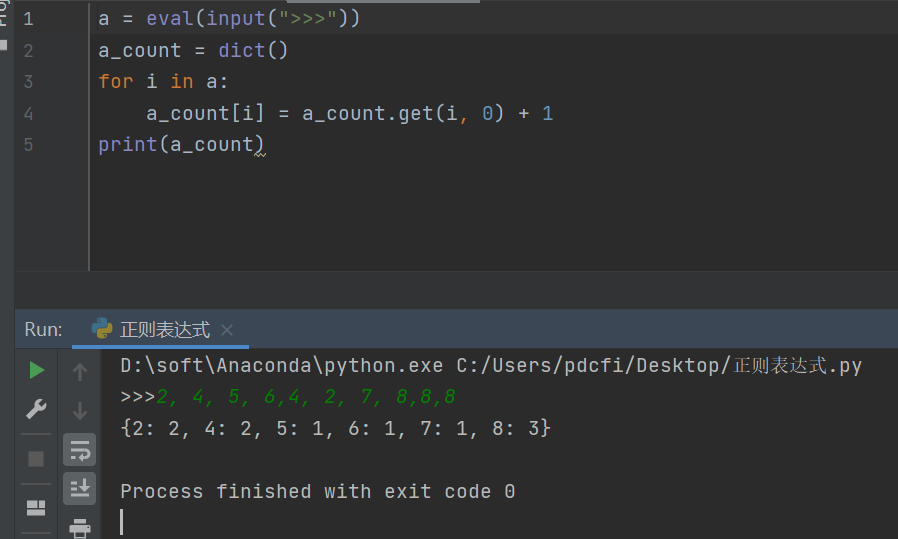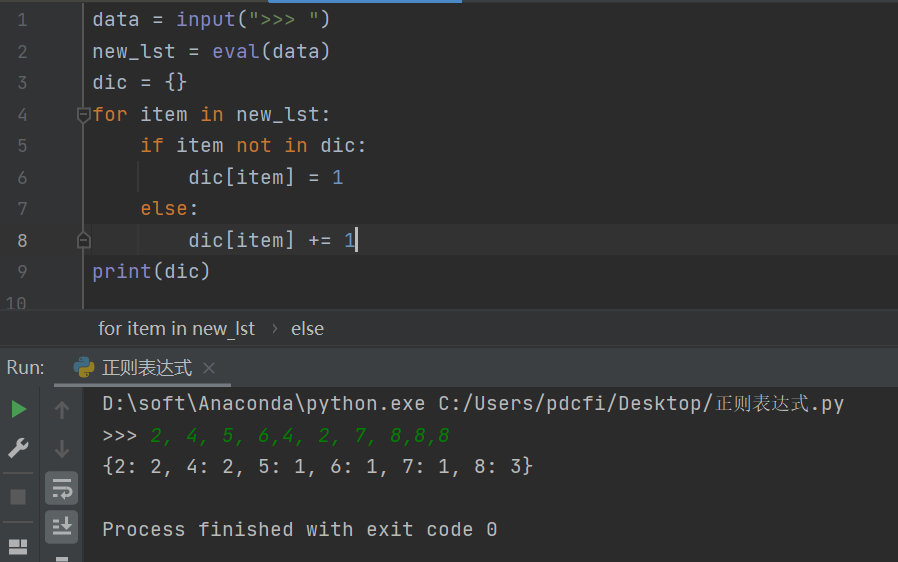# 解法一

``````import collections
data = input(">>> ")
new_lst = eval(data)
frequency = collections.Counter(new_lst)
print(frequency)
``````# 【月神】解答

``````a = eval(input(">>>"))
a_count = dict()
for i in a:
a_count[i] = a_count.get(i, 0) + 1
print(a_count)
``````# 解法三

``````data = input(">>> ")
new_lst = eval(data)
dic = {}
for item in new_lst:
if item not in dic:
dic[item] = 1
else:
dic[item] += 1
print(dic)
``````